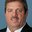Question-and-Answer Resource for the Building Energy Modeling Community
Get started with the Help page

# Modelling Air Colled Chiller: Curve of Performance and CoefficientsHello all,

Good Evening!

I found it here that to model a Chiller I would first need to get the coefficients of the curve of performance and then input these data on Open Studio. I also found here how to find the CurveFirTool, but I am not being able to get the coefficients.

1) I have tried to model an Air Colled Chiller to obtain its curve of performance and the corresponding coefficients using CurveFitTool but it is not working. As far as I am concerned I should input:

1.1) Other as DX Coil Type;

1.2) Temperature as the Independent Variable;

1.3) Biquadratic as Curve Type

1.4) SI as Units

And then, for Rated data: independent variable 1 a value of 19,4 °C, for independent variable 2 a value of 35,0 °C, for Capacity i should enter the Nominal Capacity (KW) and for Power I should enter the Power consumption (KW) related to the Nominal Capacity.

Last, for Performance Data I am entering: Independent Variable 1 as the Chilled water supply temperatura (which is fixed at 10 °C; Independent Variable 2 as the Entering Condenser Air Temperatura (which is also fixed) at 35 °C, a Part Load Capacity and its respective power consumption to complete the Performance Data.

I read that as both Independet variable 1 and 2 are fixed, I will get and error. Can someone help me with the Performance Curve and Coefficients?

The data I have includes A set of condenser inlet air temperatures - ECT (°C) and for each temperature I have the Nominal Capacity - CAP NON (KW), the Part Load Capacity - CAP (KW), and the Coefficient of Performance - COP (KW/KW). For this Chiller, the Evaporator Chilled Water Outlet is fixed at 10 (°C) and the Evaporator Water Inlet is fixed at 17 (°C).

2) Is it possible to generate the Performance Curve and get the respective coefficients from these data using CurveFitTool? If not, is there any other alternative to get these Coefficients?

3) Am I on the right path or should I use any other spreadsheet to get these results?

Thanks a lot

edit retag close merge delete

Sort by » oldest newest most votedThe Curve Fit Tool is used regularly to generate performance curve coefficients for many empirical models (e.g., coils, chillers, etc.). The data needs to include multiple data points for each independent variable. You said " The data I have includes A set of condenser inlet air temperatures - ECT (°C) and for each temperature ..." so I assume you have a set of data at only 1 leaving evaporator temperature. If this is correct, then the answer is maybe. If you have more than 5 data points (number of independent variables + 1) then you should be able to calculate the performance curve coefficients. However, the problem with this type of data set is that the coefficient for leaving evaporator water temperature will be 0. That's OK if in your simulation you ensure that the leaving evaporator water temperature always matches the temperature of your data set. If, in your simulation, the leaving evaporator water temperature deviates from the value in your data set, then your model will not include the variation in performance at that off-design temperature.

more

Hello Irebello,

I read that your units are in SI, then I understand you will need to use W not kW

more

Gotcha!

Just to verify, am I inputing the data correctly in each field? Should I really use the labels I mentioned: Other, Temperature, Biquadratic, SI?

eg. For Performance Data is Chilled Water Supply Temperature correctly imputted as Independent Variable 1? eg2. Still for Performance Data is Entering Condenser Air Temperature correctly imputted as Independent Variable 2?

And for all other input data I mentioned, did I correctly correlated the data I have to the variable in the sheet?

Best Regards

more

1

Yes, Other, Temp, BiQuad and SI are correct. Regarding data, each model has an equation for each performance curve in the Eng. Ref. For example the chiller using condenser entering fluid temperature is chilled water leaving (Tcw,l) and entering condenser fluid temp (Tcond,e):

ChillerCapFTemp = a + b (Tcw;l) + c(Tcw;l)2 + d (Tcond;e) + e(Tcond;e)2 + f (Tcw;l) (Tcond;e).

These are your data entry requirements. Also, I usually plot the data after I generate the coefficients so I know the curve works as I expect.

Another thing,

1) Why does the temperature difference between Water temperature leaving the evaporator (Tcw;l) and Water temperature entering the evaporator (Tcw;e) is not taken into account in this equation? Any ideas?

2) As it is my first time modelling a Air Cooled Chiller, may anyone check my spreadsheet just to make sure I am doing it right?

Many thanks

1) coefficient b and d already account for the absolute values of those temps, there is no need to also account for the difference (although you could include it but it's an equation for an existing model). Over-specifying the fundamental equation can lead to values of 0 for some terms. 2) I don's see a spreadsheet. You can send it to my user profile email if you like.

Once again,

Thanks a lot Richard.

Just sent you an email

Many thanks